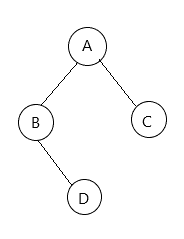• 算法思想：用一个w维护当前二叉树的宽度，使用层序遍历的方式，每次入队后更新w的值。 #include <bit/stdc++.h> using namespace std; struct TreeNode { int val; TreeNode *left, *right; }; int width...
算法思想：用一个w维护当前二叉树的宽度，使用层序遍历的方式，每次入队后更新w的值。
#include <bit/stdc++.h>
using namespace std;

struct TreeNode {
int val;
TreeNode *left, *right;
};

int width(TreeNode *root) {
if (!root) return 0;
queue<TreeNode*> q;
q.push(root);
int w = 1;// 当前二叉树的宽度为1
while (!q.empty()) {
int size = q.size();
while (size > 0) {
auto f = q.front();q.pop();
size --;
if (f->left) q.push(f->left);
if (f->right) q.push(f->right);
}
w = max(w, (int)q.size());
}
return w;
}



展开全文数据结构
• 二叉树的宽度 【问题描述】 以二叉链表为存储结构，编写算法求二叉树的宽度（具有结点数最多的那一层上的节点个数）。 【输入形式】两行，第一行是扩展二叉树的前序遍历序列。 【输出形式】二叉树的宽度。 【样例...
求二叉树的宽度
【问题描述】
以二叉链表为存储结构，编写算法求二叉树的宽度（具有结点数最多的那一层上的节点个数）。【输入形式】两行，第一行是扩展二叉树的前序遍历序列。
【输出形式】二叉树的宽度。
【样例输入】AB#D##C##
【样例输出】     2
#include<iostream>
#include<queue>
#include<deque>
using namespace std;
template<typename DataType>

struct BiNode
{
DataType data;
BiNode<DataType> *lchild,*rchild,*next;
};

template <typename DataType>
class BiTree
{
public:
BiTree()
{
root=Creat();
}
int width();
queue<BiNode<DataType>*> d;

private:
BiNode<DataType> *Creat();
BiNode<DataType> *root;
};

template<typename DataType>
BiNode<DataType> *BiTree<DataType>::Creat()
{
BiNode<DataType> *bt;
char ch;
cin>>ch;
if(ch=='#')  bt=NULL;
else
{
bt=new BiNode<DataType>;
bt->data=ch;
bt->lchild=Creat();
bt->rchild=Creat();
}
return bt;
}

template<typename DataType>
int BiTree<DataType>::width()
{

if(root == NULL)
return 0;

int maxWidth = 0;
deque<BiNode<DataType>*> d;
d.push_back(root);

while(true)
{
int len = d.size();
if(len == 0)
break;
while(len > 0)
{
BiNode<DataType> *temp = d.front();
d.pop_front();
len--;
if(temp->lchild)
d.push_back(temp->lchild);
if(temp->rchild)
d.push_back(temp->rchild);
}
maxWidth = maxWidth > d.size() ? maxWidth : d.size();

}
return maxWidth;
}

int main()
{
BiTree<char> t;
cout<<t.width()<<endl;
}




展开全文数据结构
• 二叉树的宽度：二叉树的每一层中都有一定数量的节点，节点数最多的那一层的节点数叫做二叉树的宽度。思路：递归实现。1.每个节点都可以看作根节点2.根节点(任意一个节点)的深度等于它的左子树或右子树深度最大值+13....
解释：二叉树的深度：从根结点到叶结点依次经过的结点(含根、叶结点)形成树的一条路径，最长路径的长度为树的深度。二叉树的宽度：二叉树的每一层中都有一定数量的节点，节点数最多的那一层的节点数叫做二叉树的宽度。思路：递归实现。1.每个节点都可以看作根节点2.根节点(任意一个节点)的深度等于它的左子树或右子树深度最大值+13.从根结点开始遍历，若遍历到叶子节点，深度为0//二叉树的深度public static int Depth(node root){if(root == null){return 0;}int dl = Depth(root.leftchild);int dr = Depth(root.rightchild);return dl>dr? dl+1:dr+1;}二、二叉树的宽度思路：层序遍历时添加一个计数器，记录每层的节点数1.每层出队列时记录下一层的节点数，其实就是队列的Size()2.每层遍历结束时，比较最大宽度与当前层节点数，记录最大值public static int Width(node root) {if(root == null)return 0;Queue q = new LinkedList();q.add(root);int width = 1;//最大宽度int len = 1;//当前层节点数while(q.size()>0){while(len-->0){node node = q.poll();if(node.leftchild != null){q.add(node.leftchild);}if(node.rightchild != null){q.add(node.rightchild);}}len = q.size();//每层循环结束后记录下一层的节点数width = width>q.size() ? width : q.size();}return width;}总结以上就是本文关于Java语言描述二叉树的深度和宽度的全部内容，希望对大家有所帮助。如有不足之处，欢迎留言指出。感谢朋友们对本站的支持！
展开全文• 主要介绍了C语言中计算二叉树的宽度的两种方式的相关资料,需要的朋友可以参考下
• 1. 问题描述：假设二叉树采用二叉链表存储结构存储，设计一个算法，求解出二叉树的宽度（具有节点数目最多的那一层的节点个数） 2. 思路分析： ① 首先我们可以这样想求解出二叉树中节点数目最多的那一层的节点...
1. 问题描述：假设二叉树采用二叉链表存储结构存储，设计一个算法，求解出二叉树的宽度（具有节点数目最多的那一层的节点个数）

2. 思路分析：

① 首先我们可以这样想求解出二叉树中节点数目最多的那一层的节点数目，那么我们需要求解出每一层的节点的数目是多少，取其中的最大值即可

② 但是给定的二叉树使用的是二叉链表存储结构存储的，二叉链表存储结构中存在三个变量，一个是当前节点的左孩子，一个是当前节点的右孩子，一个是当前节点的值，所以我们并不知道节点的深度，所以第一个需要解决的问题是先要对二叉树中的所有节点的深度进行初始化，我们可以才有深度优先搜索的方式来对每一个节点进行深度的初始化，所以在二叉链表的存储结构中需要维护多一个变量就是当前节点的深度，在深度优先搜索的过程中假如发现当前节点的左孩子是不为空的那么我只需要在将当前孩子的深度在当前节点的深度上加1即可，对于右孩子的深度也是一样的道理，等到深度优先搜索完成那么整棵树的节点的深度就已经初始化好了

③ 接下来就是求解出二叉树中每一层的节点数目是多少，因为借助队列对于记录每一层上的节点的数目有点不太方便，这个时候就可以使用HashMap来建立相应的映射关系，键是对应的二叉树的层次，值为二叉树层次对应的节点的数目，这样等到宽度优先搜索遍历完那么HashMap中的映射关系就建立好了

④ 对上面的HashMap中建立的映射关系进行遍历，找到二叉树中节点最多的数目是多少，也即使二叉树的宽度

3. 具体的代码如下：

import java.util.HashMap;
import java.util.LinkedList;
import java.util.Map;
import java.util.Queue;
/*
*           3
*       9       20
*     1  6    15   7
*    45  67  4
* */
public class Main {
static int k;
public static void main(String[] args) {
TreeNode<Integer> root = new TreeNode<Integer>(3);
TreeNode<Integer> l = new TreeNode<Integer>(9);
TreeNode<Integer> r = new TreeNode<Integer>(20);
TreeNode<Integer> ll = new TreeNode<Integer>(1);
TreeNode<Integer> lr = new TreeNode<Integer>(6);
TreeNode<Integer> rl = new TreeNode<Integer>(15);
TreeNode<Integer> rr = new TreeNode<Integer>(7);
TreeNode<Integer> lll = new TreeNode<Integer>(45);
TreeNode<Integer> llr = new TreeNode<Integer>(67);
TreeNode<Integer> rll = new TreeNode<Integer>(4);
root.left = l;
root.right = r;
//初始化根的层次的深度是1
root.depth = 1;
l.left = ll;
l.right = lr;
r.left = rl;
r.right = rr;
rl.left = rll;
ll.left = lll;
ll.right = llr;
initTreeNodeDepth(root);
//先序遍历查看深搜的谦虚遍历输出的结果
System.out.print("前序遍历中树节点的深度是: ");
preOrder(root);
System.out.print("\n");
System.out.print("放入到HashMap中的每一层的节点数目是: ");
int depth = treeDepth(root);
System.out.println();
System.out.println("二叉树的深度是: " + depth);
}

private static int treeDepth(TreeNode<Integer> root) {
int treedepth = 0;
Map<Integer, Integer> res = new HashMap<Integer, Integer>();
//使用层次遍历来求解出二叉树的深度
//需要借助于队列来进行
Queue<TreeNode<Integer>> queue = new LinkedList<TreeNode<Integer>>();
queue.add(root);
//将根节点的深度假如到队列中去
res.put(1, 1);
while(!queue.isEmpty()){
TreeNode<Integer> poll = queue.poll();
if(poll.left != null){
queue.add(poll.left);
//下面的if判断是为了防止空指针异常
if(res.get(poll.left.depth) == null){
res.put(poll.left.depth, 1);
}else{
res.put(poll.left.depth, res.get(poll.left.depth) + 1);
}
}
if(poll.right != null){
queue.add(poll.right);
if(res.get(poll.right.depth) == null){
res.put(poll.right.depth, 1);
}else{
res.put(poll.right.depth, res.get(poll.right.depth) + 1);
}
}
}

for(Map.Entry<Integer, Integer> map : res.entrySet()){
System.out.print(map.getValue() + " ");
if(map.getKey() > treedepth){
treedepth++;
}
}
return treedepth;
}

private static void preOrder(TreeNode<Integer> node) {
if(node == null) return;
System.out.print(node.depth + " ");
preOrder(node.left);
preOrder(node.right);
}

private static void initTreeNodeDepth(TreeNode<Integer> node) {
if(node == null) return;
//先使用深搜将所有节点的深度确定好
int depth = node.depth;
//假如当前节点的左孩子存在的话那么将左孩子的深度加1
if(node.left != null){
node.left.depth = depth + 1;
initTreeNodeDepth(node.left);
}
//假如当前节点的右孩子存在的话那么将右孩子的深度加1
if(node.right != null){
node.right.depth = depth + 1;
initTreeNodeDepth(node.right);
}
}

public static class TreeNode<T>{
TreeNode<T> left;
TreeNode<T> right;
T value;
int depth;
public TreeNode(T value) {
super();
this.value = value;
}
}
}



展开全文• 面试的时候问到了二叉树的宽度，平时做过二叉树的深度，这个其实一样，代码如下，利用层序遍历，找到每一层节点数最多的就是数的宽度 int treewidth(TreeNode *root) { if(root==NULL) { return 0; } int wid=...
•   二叉树的宽度是指二叉树各层结点个数的最大值。求二叉树的宽度可以依据与二叉树的层次遍历，我们知道，二叉树的层次遍历借助于deque实现，每次打印当前结点后将其左子树右子树入队，此时队列中既包含当前层的...
• 二叉树的宽度是指二叉树各层结点数的最大值。设有一棵二叉树，其节点值为字符型并假设各值互不相等，采用二叉链表存储表示。设计一个算法，输出该二叉树的宽度。空二叉树的宽度为0。  Input 第一行为一个整数n...
• 递归解法 ...二叉树的宽度就是二叉树宽度最大的层的宽度 def widthOfBinaryTree(self,root): self.ans = 0 dic = {} def dfs(node,pos=0,depth=0): if node: dic.setdefault(depth,pos) self.a...python
• 1. 二叉树的宽度 　若某一层的节点数不少于其他层次的节点数，那么该节点数即为二叉树的宽度。在访问过程中，我们只需要将同一层中的节点同时入栈即可。为此，我们也只需要知道上一层队列中元素的多少，在将该queue...
• 的宽度是所有层中的最大宽度。这个二叉树与满二叉树(full binary tree)结构相同，但一些节点为空。每一层的宽度被定义为两个端点(该层最左和最右的非空节点，两端点间的null节点也计入长度)之间的长度。示例 1:...
• 非递归计算二叉树的宽度 思路：用一个int型数组计算每层的宽度，然后取最大值赋予MAX并输出，那么如何改变int型数组的值便是唯一需要考虑的，上代码！ struct StNode { Node* node; int tier;//记录当前结点所在层...算法 数据结构
• 统计利用先序遍历创建的二叉树的宽度 1000(ms) 10000(kb) 2762 / 6401 利用先序递归遍历算法创建二叉树并计算该二叉树的宽度。先序递归遍历建立二叉树的方法为：按照先序递归遍历的思想将对二叉树结点的抽象访问......